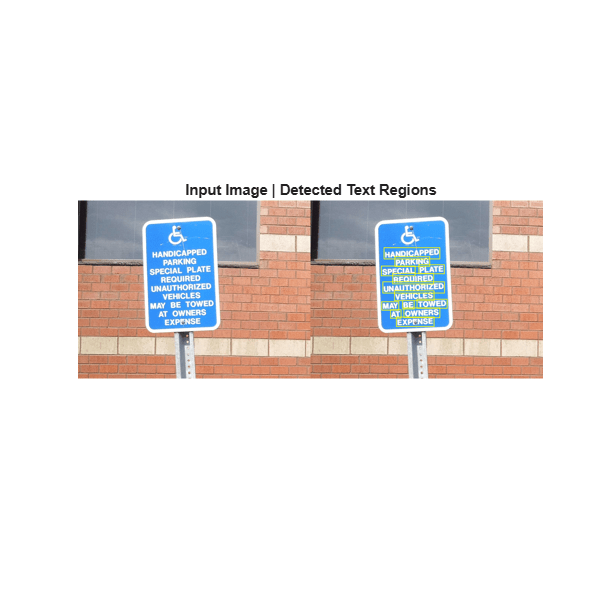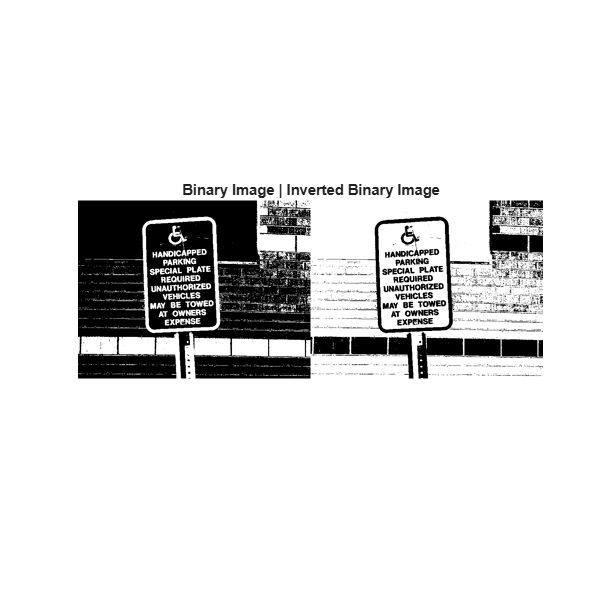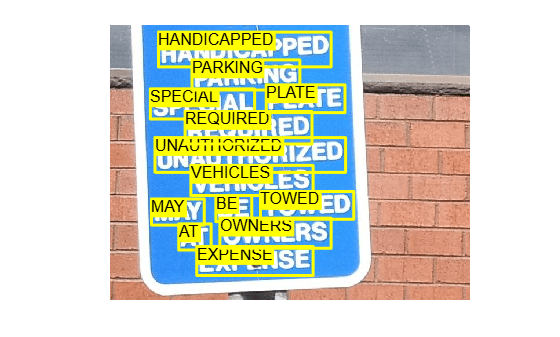# Automatically Detect and Recognize Text Using Pretrained CRAFT Network and OCR

This example shows how to perform text recognition by using the character region awareness for text detection (CRAFT) deep learning model and optical character recognition (OCR). In the example, you use a pretrained CRAFT deep learning network to detect the text regions in the input image. You can modify the region threshold and the affinity threshold values of the CRAFT model to localise an entire paragraph, a sentence or a word. Then, you use OCR to recognize the characters in the detected text regions.

Read an image into the MATLAB® workspace.

`I = imread("handicapSign.jpg");`

### Detect Text Regions

Detect text regions in the input image by using the `detectTextCRAFT` function. The `CharacterThreshold` value is the region threshold to use for localizing each character in the image. The `LinkThreshold` value is the affinity threshold that defines the score for grouping two detected texts into a single instance. You can fine-tune the detection results by modifying the region and affinity threshold values. Increase the value of the affinity threshold for more word-level and character-level detections. For information about the effect of the affinity threshold on the detection results, see the Detect Characters by Modifying Affinity Threshold example.

To detect each word on the parking sign, set the value of the region threshold to 0.3. The default value for the affinity threshold is 0.4. The output is a set of bounding boxes that localize the words in the image scene. The bounding box specifies the spatial coordinates of the detected text regions in the image.

`bbox = detectTextCRAFT(I,CharacterThreshold=0.3);`

Draw the output bounding boxes on the image by using the `insertShape` function.

`Iout = insertShape(I,"rectangle",bbox,LineWidth=4);`

Display the input image and the output text detections.

```fig = figure(Position=[1 1 600 600]); ax = gca; montage({I;Iout},Parent=ax); title("Input Image | Detected Text Regions")```### Recognize Text

Recognize the text within the bounding boxes by using the `ocr` function and display the results. The output is an `ocrText` object containing information about the recognized text, the recognition confidence, and the location of the text in the original image.

`output = ocr(I,bbox);`

Display the recognized words.

`disp([output.Words])`
``` Columns 1 through 4 {'SPECIAL'} {'MAY'} {'uniﬁfﬁoiglgen'} {'HANoIcI§I_>I_>...'} Columns 5 through 8 {'E'E_(v)U|R\E|3'} {'VEHICLES'} {'FARKING'} {'EXPENSE'} Columns 9 through 11 {'‘owuens'} {'TOWED'} {'PLATE'} ```

### Analyze Recognition Results

Out of 13 words on the parking sign only 6 words are recognized correctly. The words `UNAUTHORIZED`, `HANDICAPPED`, `REQUIRED`, `PARKING`, `BE`, and `OWNERS` are not recognized correctly. The performance of the OCR method depend on the text detection results and the characteristics of the image background. As a preprocessing step, the `ocr` function performs binarization to segment the text regions from the background. For good segmentation results, the image background must be uniform and the text regions must have high contrast over the background. Otherwise, the segmented text regions might have outliers which in turn affect the recognition results.

You can use `imbinarize` function to check the initial binarization step because both `ocr` and the default '`global`' method in `imbinarize` use Otsu's method for image binarization.

```Idet = cell(1,size(bbox,1)); Iseg = cell(1,size(bbox,1)); for i=1:size(bbox,1) roi = bbox(i,:); Idet{i} = I(roi(2):roi(2)+roi(4),roi(1):roi(1)+roi(3),:); Iseg{i} = imbinarize(rgb2gray(Idet{i})); end```

Display the text detections obtained using `detectTextCRAFT` function and the corresponding segmentation results. You can notice that the segmented text regions corresponding to the words `UNAUTHORIZED`, `HANDICAPPED`, and `REQUIRED` contain outliers.

```fig1 = figure; set(fig1,Position=[1 1 900 400]) hPanel1 = uipanel(fig1,Position=[0 0 0.5 1]); hPlot1 = axes(hPanel1); hPanel2 = uipanel(fig1,Position=[0.5 0 0.5 1]); hPlot2 = axes(hPanel2); montage(Idet,Parent=hPlot1) montage(Iseg,Parent=hPlot2) title("Segmented Text Regions",Parent=hPlot2)```### Improve Recognition Results

To improve the recognition results, preprocess the detected text regions so that the input to `ocr` is well localized detections and have no outliers.

Reduce the number of outliers in the segmented text regions by computing tightly localized detections using the `detectTextCRAFT` function. Increase the value of the region threshold to reduce the number of false detections and compute bounding boxes that tightly localize the text regions in the image. Set the value of `CharacterThreshold` parameter to 0.55 and compute the text detections. The default value for the affinity threshold is 0.4.

`newBbox = detectTextCRAFT(I,CharacterThreshold=0.55);`

Draw the output bounding boxes on the image by using the `insertShape` function. Display the detection results. You can notice that the text regions in the image are tightly localized.

```Iout = insertShape(I,"rectangle",newBbox,LineWidth=3); figure imshow(Iout) title("Detected Text Regions for Region Threshold = 0.55")```Recognize the text within the bounding boxes by using the `ocr` function and display the recognized words.

```output = ocr(I,newBbox); disp([output.Words])```
``` Columns 1 through 4 {'SPECIAL'} {'UNAUTHORIZED'} {'HANDICAPPED'} {'REQUIRED'} Columns 5 through 8 {'VEHICLES'} {'PARKING'} {'EXPENSE'} {'PLATE'} ```

The words on the parking sign are correctly recognized except for these small words: `MAY`, `BE`, and `AT`. This is because the bounding box is too tight for OCR to detect small length words.

Follow these preprocessing steps to further improve the recognition accuracy.

• Adjust the contrast of the detected text regions by using `imadjust` function. Contrast enhancement improves segmentation accuracy when the input is a low contrast image.

• Segment the text regions from the image background by using `imbinarize` function. You can also use other segmentation methods like k-means clustering or adaptive thresholding based on the complexity of the image scene.

• Pad the image by adding more pixels along the image boundary. If the intensity value of the foreground text region is `1` then the padding pixel value must be `0` and vice-versa.

• Perform morphological erosion to remove small outliers in the segmented text region, if any.

Recognize the text within the bounding boxes by using the `ocr` function. To remove blank spaces, use `deblank` function.

```Icorrect = cell(1,size(newBbox,1)); finalOutput = cell(1,size(newBbox,1)); recognizedWords = cell(1,size(newBbox,1)); for i=1:size(newBbox,1) roi = newBbox(i,:); Icrop = I(roi(2):roi(2)+roi(4),roi(1):roi(1)+roi(3),:); Ipreprocess = rgb2gray(Icrop); Ipreprocess = imadjust(Ipreprocess); Isegment = imbinarize(Ipreprocess); Isegment = padarray(Isegment,[15 15],0,'both'); se = strel('square',2); Icorrect{i} = imerode(Isegment,se); finalOutput{i} = ocr(Icorrect{i}); recognizedWords{i} = [deblank(finalOutput{i}.Text)]; end```

Display the segmentation results. Now the text detections are tightly localized, the segmented texts does not have any outliers. The area of the segmented text regions has also increased because of padding.

```figure(Position=[1 1 400 400]); ax3 = gca; montage(Icorrect,Parent=ax3) title("Segmented Text Regions")```Display the results and annotate the recognized words on the parking sign.

`disp(recognizedWords)`
``` Columns 1 through 5 {'SPECIAL'} {'MAY'} {'UNAUTHORIZED'} {'HANDICAPPED'} {'AT'} Columns 6 through 10 {'REQUIRED'} {'VEHICLES'} {'PARKING'} {'EXPENSE'} {'BE'} Columns 11 through 13 {'OWNERS'} {'TOWED'} {'PLATE'} ```
```Iannotate = I; for cnt = 1:size(finalOutput,2) if ~isempty(finalOutput{cnt}.Words) Iannotate = insertObjectAnnotation(Iannotate,"Rectangle",newBbox(cnt,:),finalOutput{cnt}.Words,FontSize=18); end end figure imshow(Iannotate)```